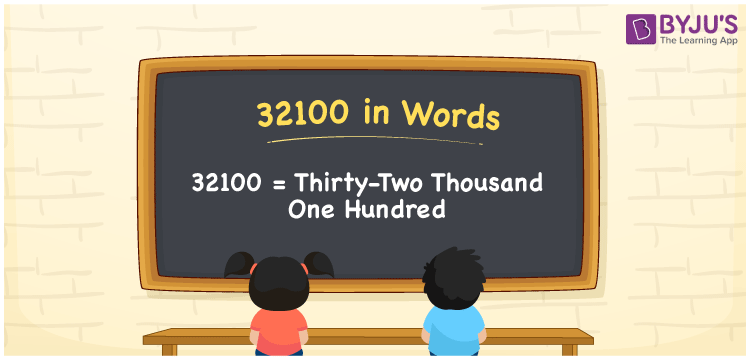# 32100 in Words

32100 in words can be written as Thirty-Two Thousand One Hundred. If you buy utensils for the kitchen for Rs. 32100, then you can say that “I bought utensils for the kitchen for Thirty-Two Thousand One Hundred Rupees”. The numbers in words concept is explained in a simple language to help students grasp it. Therefore, 32100 can be read as “Thirty-Two Thousand One Hundred” in words.

 32100 in words Thirty-Two Thousand One Hundred Thirty-Two Thousand One Hundred in Numbers 32100

## 32100 in English Words## How to Write 32100 in Words?

The concept of place value chart and the expanded form of the number 32100 can be learnt easily by the students in this section.

 Ten Thousands Thousands Hundreds Tens Ones 3 2 1 0 0

32100 in expanded form is:

3 x Ten Thousand + 2 x Thousand + 1 × Hundred + 0 × Ten + 0 × One

= 3 x 10000 + 2 x 1000 + 1 × 100 + 0 × 10 + 0 × 1

= 30000 + 2000 + 100

= 32100

= Thirty-Two Thousand One Hundred

Therefore, 32100 in words is written as Thirty-Two Thousand One Hundred.

32100 is a natural number that precedes 32101 and succeeds 32099.

32100 in words – Thirty-Two Thousand One Hundred

Is 32100 an odd number? – No

Is 32100 an even number? – Yes

Is 32100 a perfect square number? – No

Is 32100 a perfect cube number? – No

Is 32100 a prime number? – No

Is 32100 a composite number? – Yes

## Frequently Asked Questions on 32100 in Words

Q1

### Write 32100 in words.

32100 can be written in words as “Thirty-Two Thousand One Hundred”.
Q2

### How do you write Thirty-Two Thousand One Hundred in numbers?

Thirty-Two Thousand One Hundred can be written in numbers as 32100.
Q3

### Is 32100 an even number?

Yes, 32100 is an even number as it is completely divisible by 2.